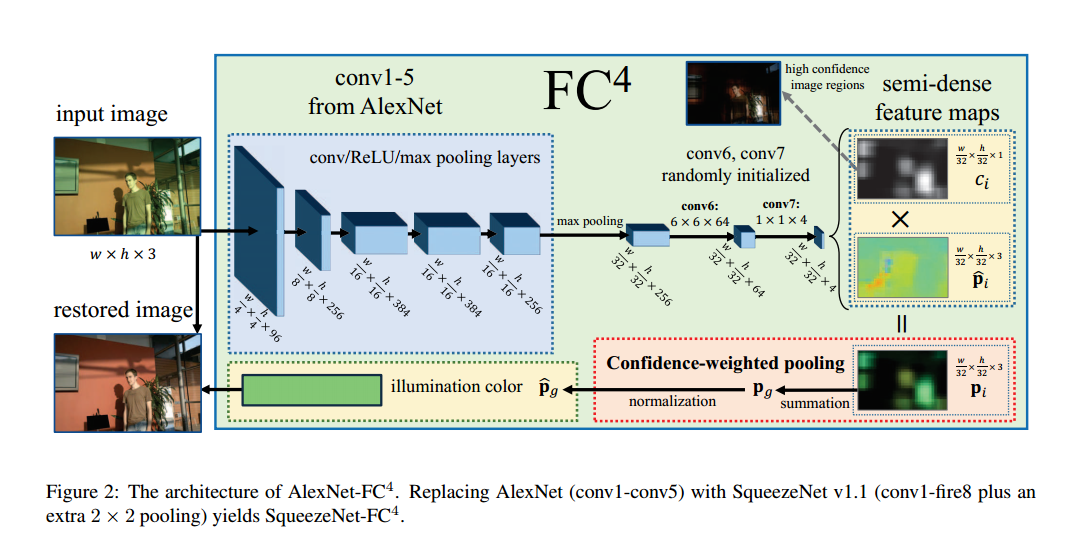#### Introduction

This paper comes from 2017 CVPR,which introduce a confidence-weighted pooling for color constancy. The confidence weights are learned and applied within a pooling layer where the local estimates are merged into a global solution. Breifly,I think the network aims to get the semantic information thus can be used for which local areas in an image are informative for color constancy.The advantages of this network can be attributed as follows:
1.This network can distinguish useful and noisy data.
2.End-to-end training, direct processing of images with arbitrary size, and much faster computation.
3.Less prone to large estimation errors.

statistics-based and learning-based methods,such as patch-based cnn…….

#### Main Work

The goal is to estimate the global illumination color $p_g=(r,g,b)$ so that its color can be removed from the image,by replacing the normalized illumination color with a canonical light source color, usually pure white. So the goal is to minimize the loss function:

where the $\hat{p_g}$ is the estimate map and $\hat{p_g^*}$ is the groundtruth. Since (1) output the estimate of the local region,suppose the R = {R1, R2, . . . , Rn} is a set of overlapping local regions in I, and function $g(R_i)$ outputs the regional light color estimate for $R_i$,the final result is:

where $c(R_i)$ is a weighting function that represents the confidence value of $R_i$. Intuitively, if $R_i$ is a local region that contains useful semantic context for illumination estimation, then $c(R_i)$ should be large.The total architecture can be shown as follows:##### Fully Convolutional Architecture

Unlike previous patch-based methods,this paper treat each $R_i$ independently over an image and use a CNN to learn g,so as to get their relative importance for estimating the global illustration,thus given an image, we wish to determine the local estimates simultaneously. In this paper,it adopt a pretrained Alexnet as the default FCN architecture.
The network output the conv7 layer as the 4 channel feature map,and force the first three channel feature map represent the color triplet $\hat{p_i}=g(R_i)$ estimated from each corresponding patch,while the last one represent its confidence $c_i = c(R_i)$,than the fourchannels are passed through a ReLU layer to avoid negtive values,and it can define the weighted estimate $p_i$ as $c_i p^i$.

##### Confidence-weighted Pooling Layer

As different local regions may differ in value for illumination estimation based on their semantic content.It should be noticed that during back-propagation, this pooling layer serves as a “gradient dispatcher” which backpropagates gradients to local regions with respect to their confidence.So it means that the gradients should have the same direction but have different magnitudes that are proportional to $c_i$,finally for confidence $c_i$,we have:

Intuitively, as long as a local estimate helps the global estimation get closer to the ground truth, the network increases the corresponding confidence.

……

translation:
1.triplet:三重态

0%